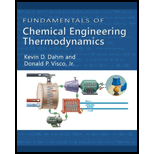# Steam enters an adiabatic, steady-state turbine at T = 300°C and P = 5 bar. The effluent from the turbine has P = 1 bar, and is a liquid–vapor mixture with q = 0.9. A. Find the difference between H ^ of the exiting stream and H ^ of the entering stream. B. In order to produce 100 MJ/min of work, what is the required flow rate of the entering stream?### Fundamentals of Chemical Engineeri...

1st Edition
Kevin D. Dahm + 1 other
Publisher: Cengage Learning
ISBN: 9781111580704### Fundamentals of Chemical Engineeri...

1st Edition
Kevin D. Dahm + 1 other
Publisher: Cengage Learning
ISBN: 9781111580704

#### Solutions

Chapter
Section
Chapter 3.8, Problem 7E
Textbook Problem

## Steam enters an adiabatic, steady-state turbine at T = 300°C and P = 5 bar. The effluent from the turbine has P = 1 bar, and is a liquid–vapor mixture with q = 0.9.A. Find the difference between H ^ of the exiting stream and H ^ of the entering stream.B. In order to produce 100 MJ/min of work, what is the required flow rate of the entering stream?

Expert Solution

(A)

Interpretation Introduction

Interpretation:

The difference between specific enthalpy of exiting and entering stream of the steam.

Concept Introduction:

The exiting specific enthalpy (H^exiting) equation.

H^exiting=(1x)H^L+xH^V

Here, enthalpy in vapor phase is H^V, quality or dryness fraction is x, and enthalpy in liquid phase is H^L.

The difference between specific enthalpy (ΔH^) of exiting and entering stream of the steam.

ΔH^=H^exitingH^entering

Here, entering enthalpy is H^entering and exiting enthalpy is H^exiting.

### Explanation of Solution

Given information:

Refer appendix table A-4, “Saturated steam-Compressed liquid”, obtain the value of entering specific enthalpy of saturated liquid phase at a pressure of 5bar.

H^entering=640.1kJ/kg

Refer appendix table A-1, “Saturated steam-Pressure increments”, obtain the value of specific enthalpy in vapor phase at a pressure of 1bar.

H^V=2674.9kJ/kg

Refer appendix table A-1, “Saturated steam-Pressure increments”, obtain the value of specific enthalpy in liquid phase at a pressure of 1bar.

H^L=417.5kJ/kg

Write the expression to calculate the exiting specific enthalpy (H^exiting) of the steam.

H^exiting=(1x)H^L+xH^V        (1)

Substitute 0.9 for x, 417.5kJ/kg for H^L, and 2674.9kJ/kg for H^V in Equation (1).

H^exiting=(10

Expert Solution

(B)

Interpretation Introduction

Interpretation:

Mass flow rate (m˙) of the entering steam.

Concept Introduction:

Work done equation for the adiabatic turbine.

W˙m˙=H^exitingH^entering

### Want to see the full answer?

Check out a sample textbook solution.See solution

### Want to see this answer and more?

Bartleby provides explanations to thousands of textbook problems written by our experts, many with advanced degrees!

See solution

Find more solutions based on key concepts
Show solutions
What is an assistive technology system?

Fundamentals of Information Systems

For Problems 3.30 through 3.40, you are asked to look up some examples of specific standardsand codes that are ...

Engineering Fundamentals: An Introduction to Engineering (MindTap Course List)

What is a VPN? Why is it becoming more widely used?

Principles of Information Security (MindTap Course List)

What does NFPA stand for?

Precision Machining Technology (MindTap Course List)

In addition to sharing online calendars, is it possible to share calendars you create in programs such as Micro...

Enhanced Discovering Computers 2017 (Shelly Cashman Series) (MindTap Course List)

If your motherboard supports ECC DDR3 memory, can you substitute non-ECC DDR3 memory?

A+ Guide to Hardware (Standalone Book) (MindTap Course List)